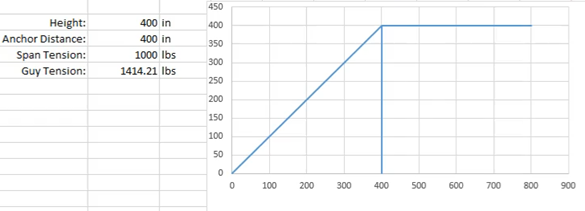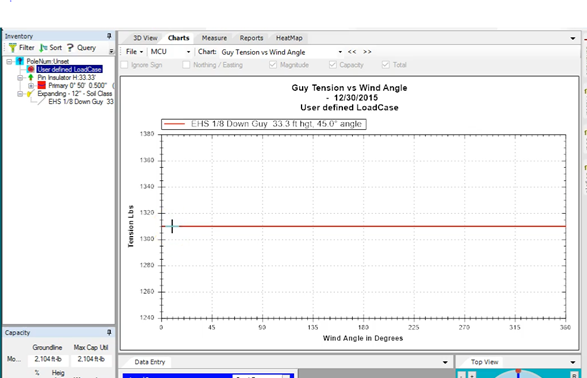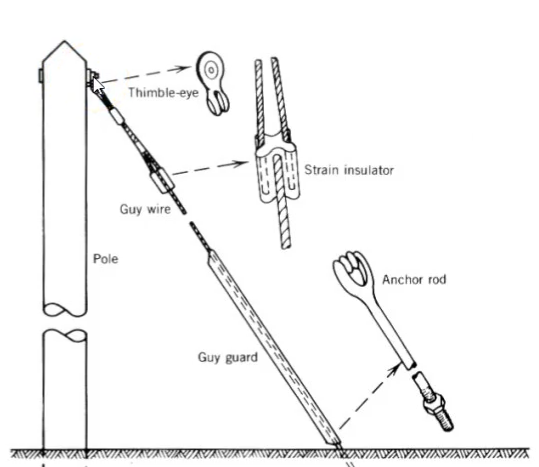## Differences between O-Calc and hand calculations

When comparing O-Calc to simplified hand calculations it is sometimes surprising to find that there can be some differences.

Let’s look at a very simple example of a dead-end load of 1000 lbs applied 400 inches above the ground guyed by a down guy that is 180 degrees out of phase and with both the guy height and the anchor distance at 400 inches so that the guy declination angle is exactly 45 degrees. This is one of the simplest possible examples as we can hand calculate the expected guy tension with ease as a function of the tension and the cosine of the declination.When we set up a similar pole in O-Calc with the wind set to 0 psf and similar tension we are surprised to see that the resulting guy tension is nearly 100 lbs less!There are two reasons for this:

1) In O-Calc the pole itself is an active part of the calculation. When the tension is applied it is deflecting the pole as well as elongating the down guy. As a result some of the span tension is being counteracted by the deformation of the pole itself!

2) O-Calc is actually modelling the geometry of the pole and the guy. Rather than applying the guying force at the exact end of the load (the center of the pole) it applies it at the face of the pole via the guy hook. As a result there is an additional lever effect transforming some of the vertical component of guying force into effective horizontal offsetting load.When both of these factors are compensated for by altering the pole geometry and the elastic modulus of the wood so that the pole provides no lever force counteracting the span’s tension we get the same value as the hand calculations.

The following vide explains these differences in more detail:

In conclusion the differences between O-Calc results and simplified hand calculations result from exactly that, the hand calculations are simplified where the O-Calc system models geometries and forces to a level that would make hand calculations impossible.